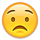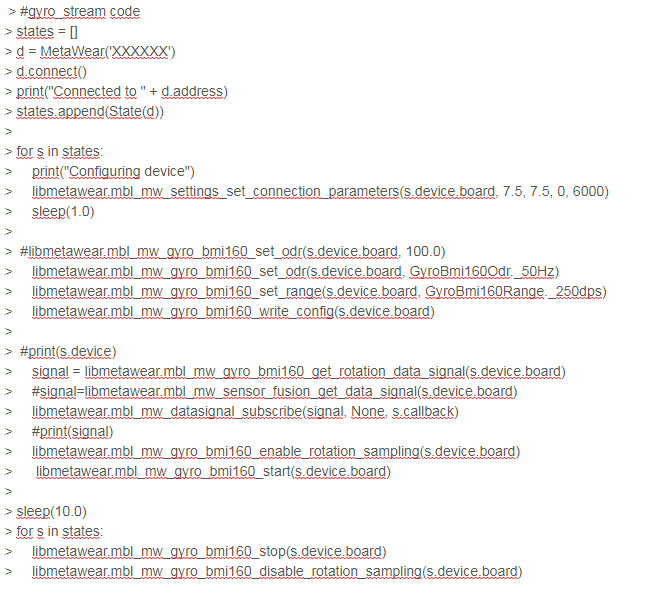# MetamotionR Signal Processing

in Python, I cannot process the data I have received from the sensor(MetamotionR). With parse_value(data) I can show it but not processing. Is there any suggestion?

• Post the code you are currently working with
• Describe what is wrong with it; post output logs
• Describe the expected behavior
• modify of acc_stream code[examples]

``````def __init__(self, device):
self.device = device
self.samples = 0
self.callback = FnVoid_VoidP_DataP(self.data_handler)

def data_handler(self, csv, data):

datax=parse_value(data).x
datay=parse_value(data).y
dataz=parse_value(data).z
``````

In here, my aim take the rotation data on the gyroscope sensor. for this, I try to get the data in the above code but it doesnt directly involve rotation information, just to change in rotation. Is there a any code that is directly rotation data?

• This is in our matlab tutorial but it is in python (this examples stream quaternion data into matlab): https://github.com/mbientlab/MetaWear-Tutorial-Matlab/blob/master/echo_quat_server.py

• def data_handler(self, csv, data):
datax=parse_value(data).x
datay=parse_value(data).y
dataz=parse_value(data).z

I have noticed that the data I have received does not include x, y, and z angle information. Is there any suggestion i?

• Laura ? Eric?• *

• # gyro_stream code

states = []
d = MetaWear('XXXXXX')
d.connect()
states.append(State(d))

for s in states:
print("Configuring device")
libmetawear.mbl_mw_settings_set_connection_parameters(s.device.board, 7.5, 7.5, 0, 6000)
sleep(1.0)

#libmetawear.mbl_mw_gyro_bmi160_set_odr(s.device.board, 100.0)
libmetawear.mbl_mw_gyro_bmi160_set_odr(s.device.board, GyroBmi160Odr._50Hz)
libmetawear.mbl_mw_gyro_bmi160_set_range(s.device.board, GyroBmi160Range._250dps)
libmetawear.mbl_mw_gyro_bmi160_write_config(s.device.board)

#print(s.device)
signal = libmetawear.mbl_mw_gyro_bmi160_get_rotation_data_signal(s.device.board)
#signal=libmetawear.mbl_mw_sensor_fusion_get_data_signal(s.device.board)
libmetawear.mbl_mw_datasignal_subscribe(signal, None, s.callback)
#print(signal)
libmetawear.mbl_mw_gyro_bmi160_enable_rotation_sampling(s.device.board)
libmetawear.mbl_mw_gyro_bmi160_start(s.device.board)

sleep(10.0)
for s in states:
libmetawear.mbl_mw_gyro_bmi160_stop(s.device.board)
libmetawear.mbl_mw_gyro_bmi160_disable_rotation_sampling(s.device.board)

result: change:x axis 90 degrees

{x : 0.320, y : 0.328, z : -0.480}
{x : 0.373, y : 0.229, z : -0.488}
{x : 0.373, y : 0.305, z : -0.526}
{x : 0.305, y : 0.328, z : -0.526}
{x : 0.328, y : 0.335, z : -0.595}
{x : 0.320, y : 0.282, z : -0.549}
{x : -0.831, y : -0.015, z : -7.79}
{x : -6.364, y : -2.439, z : -83.7}
{x : 5.800, y : -1.456, z : -103.2}
{x : 1.082, y : -1.662, z : -76.50}
{x : -6.928, y : 10.305, z : -17.9}

when I run the code,I cannot see any reasonable change on the result. I cannot understand what is the wrong

.

• M_data=parse_value(data,n_elem = 2)
in here, I cannot understand n_elem=1 or 2.

• edited March 2019
1. Wrap your code blocks with ```. What you posted is unreadable.
2. Explain what is wrong with your code. What is the expected behavior? What is the actual behavior?
•*expected behavior:When I rotate the sensor 90 degrees in any axis, I want to see see this angle on the data I received from the sensor.
*actual behavior:When I rotate the sensor any axis, I cannot see proper value on the data.
*I think this code doesn't get the proper data through the sensor. I hope I can describe the problem properly.

• edited March 2019

I'll say it once again, wrap your code blocks with ```.

Use sensor fusion. Read the links Laura and I provided in our previous posts.

• edited March 2019

You are streaming gyro data which is angular velocity and not an angle in degrees. If you want vectors, use sensor fusion.

• I used Sensor Fusion, but I have a problem. When I first ran the code, this code calculated the value correctly. Now, the code is running but cannot calculate the values. Is there any suggestion in this problem?

• @Bacaksiz_1907 said:
I used Sensor Fusion, but I have a problem. When I first ran the code, this code calculated the value correctly. Now, the code is running but cannot calculate the values. Is there any suggestion in this problem?

Repeating myself again....

@Eric said:

• Post the code you are currently working with
• Describe what is wrong with it; post output logs
• Describe the expected behavior